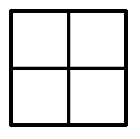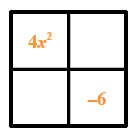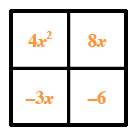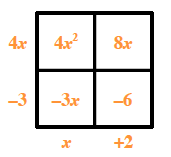### Home > CAAC > Chapter 10 > Lesson 10.2.3 > Problem10-75

10-75.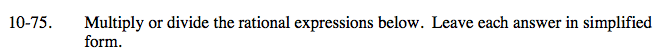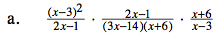$\frac{(x-3)(x-3)(2x-1)(x+6)}{(2x-1)(3x-14)(x+6)(x-3)}$

$\frac{(x-3)}{(x-3)}=\frac{(2x-1)}{(2x-1)}=\frac{(x+6)}{(x+6)}=1$

$\frac{(x-3)}{(3x-14)}$

Multiply across the numerator and denominator.

Look for factors that simplify to one.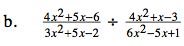$\frac{(4x^{2}+5x-6)}{(3x^{2}+5x-2)}\cdot \frac{(6x^{2}-5x+1)}{(4x^{2}+x-3)}$

Use a generic rectangle to factor each trinomial.

4x2 + 5x − 6

Put the first and last terms in opposite corners.

The products of the diagonals need to be equal. In this case find two (missing) terms whose product is −24x2. For this expression their sum must be 5x.

Write the missing terms in the blank corners of the rectangle.

Find the greatest common factor of each row and column. Write the factors on the outside of the rectangle.

The side 'lengths' of the rectangle are the factors of the trinomial.

4x2 + 5x − 6 = (4x − 3)(x + 2)

You can use this method to factor the other three trinomials. Then, complete the division problem.

Convert the quotient to a product.